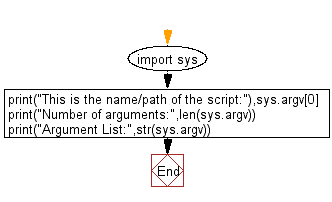﻿ Python: Get the command-line arguments passed to a script - w3resource# Python: Get the command-line arguments passed to a script

## Python Basic: Exercise-76 with Solution

Write a Python program to get the command-line arguments (name of the script, the number of arguments, arguments) passed to a script.

Sample Solution:

Python Code (test.py):

``````import sys
print("This is the name/path of the script:"),sys.argv
print("Number of arguments:",len(sys.argv))
print("Argument List:",str(sys.argv))
```
```

The command executed in command prompt:

``````[email protected]:~\$ python test.py arg1 arg2 arg3
``````

Sample Output:

```This is the name/path of the script: test.py
('Number of arguments:', 4)
('Argument List:', "['test.py', 'arg1', 'arg2', 'arg3']")
```

Flowchart:Have another way to solve this solution? Contribute your code (and comments) through Disqus.

What is the difficulty level of this exercise?

Test your Python skills with w3resource's quiz

﻿

## Python: Tips of the Day

Unpack variables from iterable:

```# One can unpack all iterables (tuples, list etc)
>>> a, b, c = 1, 2, 3
>>> a, b, c
(1, 2, 3)

>>> a, b, c = [1, 2, 3]
>>> a, b, c
(1, 2, 3)
```